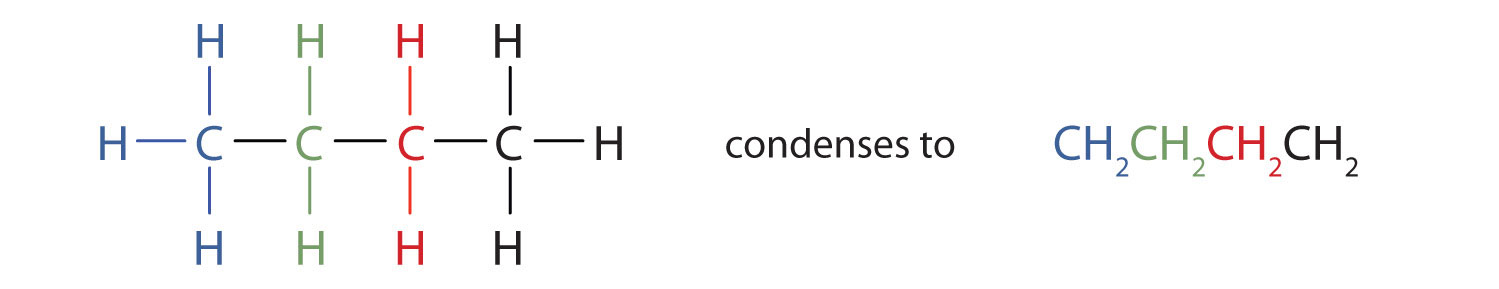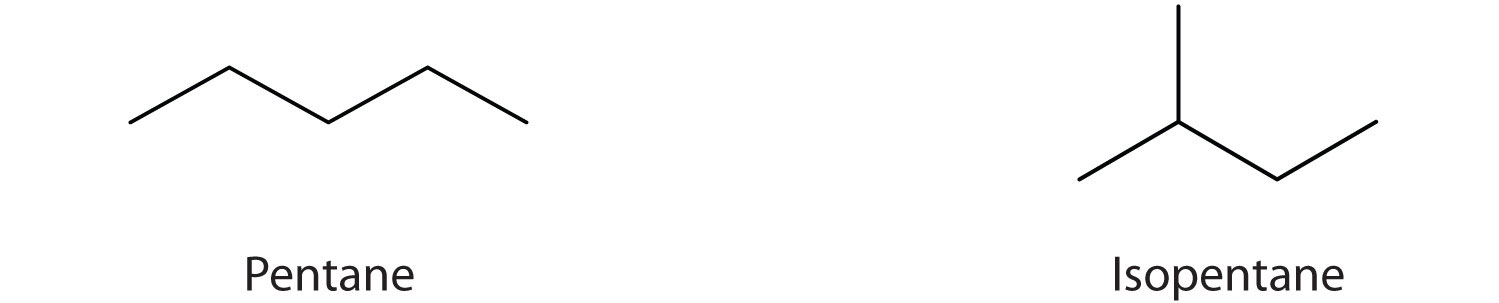This is “Condensed Structural and Line-Angle Formulas”, section 12.4 from the book Introduction to Chemistry: General, Organic, and Biological (v. 1.0). For details on it (including licensing), click here.

Has this book helped you? Consider passing it on:
Creative Commons supports free culture from music to education. Their licenses helped make this book available to you.
DonorsChoose.org helps people like you help teachers fund their classroom projects, from art supplies to books to calculators.

## 12.4 Condensed Structural and Line-Angle Formulas

### Learning Objectives

1. Write condensed structural formulas for alkanes given complete structural formulas.
2. Draw line-angle formulas given structural formulas.

We use several kinds of formulas to describe organic compounds. A molecular formula shows only the kinds and numbers of atoms in a molecule. For example, the molecular formula C4H10 tells us there are 4 carbon atoms and 10 hydrogen atoms in a molecule, but it doesn’t distinguish between butane and isobutane. A structural formulaA chemical formula that shows how the atoms of a molecule are attached to one another. shows all the carbon and hydrogen atoms and the bonds attaching them. Thus, structural formulas identify the specific isomers by showing the order of attachment of the various atoms.

Unfortunately, structural formulas are difficult to type/write and take up a lot of space. Chemists often use condensed structural formulasAn organic chemical formula that shows the hydrogen atoms (or other atoms or groups) right next to the carbon atoms to which they are attached. to alleviate these problems. The condensed formulas show hydrogen atoms right next to the carbon atoms to which they are attached, as illustrated for butane:The ultimate condensed formula is a line-angle formulaAn organic chemical formula in which carbon atoms are implied at the corners and ends of lines. Each carbon atom is understood to be attached to enough hydrogen atoms to give each carbon atom four bonds., in which carbon atoms are implied at the corners and ends of lines, and each carbon atom is understood to be attached to enough hydrogen atoms to give each carbon atom four bonds. For example, we can represent pentane (CH3CH2CH2CH2CH3) and isopentane [(CH3)2CHCH2CH3] as follows:### Note

Parentheses in condensed structural formulas indicate that the enclosed grouping of atoms is attached to the adjacent carbon atom.

### Key Takeaways

• Condensed chemical formulas show the hydrogen atoms (or other atoms or groups) right next to the carbon atoms to which they are attached.
• Line-angle formulas imply a carbon atom at the corners and ends of lines. Each carbon atom is understood to be attached to enough hydrogen atoms to give each carbon atom four bonds.

### Exercises

1. Write the condensed structural formula for each structural formula.

1.2.3.2. A condensed structural formula for isohexane can be written as (CH3)2CHCH2CH2CH3. Draw the line-angle formula for isohexane.

3. Draw a line-angle formula for the compound CH3CH2CH(CH3)CH2CH2CH3.

4. Give the structural formula for the compound represented by this line-angle formula:1.